The CORR Procedure
 PROC CORR Statement

PROC CORR <options> ;
Table 2.1 summarizes the options available in the PROC CORR statement.
Table 2.1 Summary of PROC CORR Options

Option

Description

Data Sets

DATA=

Specifies the input data set

OUTH=

Specifies the output data set with Hoeffding’sstatistics

OUTK=

Specifies the output data set with Kendall correlation statistics

OUTP=

Specifies the output data set with Pearson correlation statistics

OUTS=

Specifies the output data set with Spearman correlation statistics

Statistical Analysis

EXCLNPWGT

Excludes observations with nonpositive weight values from the analysis

FISHER

Requests correlation statistics using Fisher’stransformation

HOEFFDING

Requests Hoeffding’s measure of dependence,KENDALL

Requests Kendall’s tau-b

NOMISS

Excludes observations with missing analysis values from the analysis

PEARSON

Requests Pearson product-moment correlation

SPEARMAN

Requests Spearman rank-order correlation

Pearson Correlation Statistics

ALPHA

Computes Cronbach’s coefficient alpha

COV

Computes covariances

CSSCP

Computes corrected sums of squares and crossproducts

FISHER

Computes correlation statistics based on Fisher’stransformation

SINGULAR=

Specifies the singularity criterion

SSCP

Computes sums of squares and crossproducts

VARDEF=

Specifies the divisor for variance calculations

ODS Output Graphics

PLOTS=MATRIX

Displays the scatter plot matrix

PLOTS=SCATTER

Displays scatter plots for pairs of variables

Printed Output

BEST=

Displays the specified number of ordered correlation coefficients

NOCORR

Suppresses Pearson correlations

NOPRINT

Suppresses all printed output

NOPROB

Suppresses-values

NOSIMPLE

Suppresses descriptive statistics

RANK

Displays ordered correlation coefficients

The following options can be used in the PROC CORR statement. They are listed in alphabetical order.

ALPHA

calculates and prints Cronbach’s coefficient alpha. PROC CORR computes separate coefficients using raw and standardized values (scaling the variables to a unit variance of 1). For each VAR statement variable, PROC CORR computes the correlation between the variable and the total of the remaining variables. It also computes Cronbach’s coefficient alpha by using only the remaining variables.

If a WITH statement is specified, the ALPHA option is invalid. When you specify the ALPHA option, the Pearson correlations will also be displayed. If you specify the OUTP= option, the output data set also contains observations with Cronbach’s coefficient alpha. If you use the PARTIAL statement, PROC CORR calculates Cronbach’s coefficient alpha for partialled variables. See the section Partial Correlation for details.

BEST=n

prints thehighest correlation coefficients for each variable,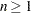. Correlations are ordered from highest to lowest in absolute value. Otherwise, PROC CORR prints correlations in a rectangular table, using the variable names as row and column labels.

If you specify the HOEFFDING option, PROC CORR displays thestatistics in order from highest to lowest.

COV

displays the variance and covariance matrix. When you specify the COV option, the Pearson correlations will also be displayed. If you specify the OUTP= option, the output data set also contains the covariance matrix with the corresponding _TYPE_ variable value 'COV.' If you use the PARTIAL statement, PROC CORR computes a partial covariance matrix.

CSSCP

displays a table of the corrected sums of squares and crossproducts. When you specify the CSSCP option, the Pearson correlations will also be displayed. If you specify the OUTP= option, the output data set also contains a CSSCP matrix with the corresponding _TYPE_ variable value 'CSSCP.' If you use a PARTIAL statement, PROC CORR prints both an unpartial and a partial CSSCP matrix, and the output data set contains a partial CSSCP matrix.

DATA=SAS-data-set

names the SAS data set to be analyzed by PROC CORR. By default, the procedure uses the most recently created SAS data set.

EXCLNPWGT
EXCLNPWGTS

excludes observations with nonpositive weight values from the analysis. By default, PROC CORR treats observations with negative weights like those with zero weights and counts them in the total number of observations.

FISHER <( fisher-options )>

requests confidence limits and-values under a specified null hypothesis,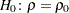, for correlation coefficients by using Fisher’stransformation. These correlations include the Pearson correlations and Spearman correlations.

The following fisher-options are available:

ALPHA=specifies the level of the confidence limits for the correlation,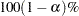. The value of the ALPHA= option must be between 0 and 1, and the default is ALPHA=0.05.

specifies whether or not the bias adjustment is used in constructing confidence limits. The BIASADJ=YES option also produces a new correlation estimate that uses the bias adjustment. By default, BIASADJ=YES.

RHO0=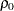specifies the valuein the null hypothesis, where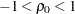. By default, RHO0=0.

TYPE=LOWER | UPPER | TWOSIDED

specifies the type of confidence limits. The TYPE=LOWER option requests a lower confidence limit from the lower alternative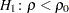, the TYPE=UPPER option requests an upper confidence limit from the upper alternative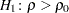, and the default TYPE=TWOSIDED option requests two-sided confidence limits from the two-sided alternative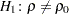.

HOEFFDING

requests a table of Hoeffding’sstatistics. Thisstatistic is 30 times larger than the usual definition and scales the range between0.5 and 1 so that large positive values indicate dependence. The HOEFFDING option is invalid if a WEIGHT or PARTIAL statement is used.

KENDALL

requests a table of Kendall’s tau-b coefficients based on the number of concordant and discordant pairs of observations. Kendall’s tau-b ranges from1 to 1.

The KENDALL option is invalid if a WEIGHT statement is used. If you use a PARTIAL statement, probability values for Kendall’s partial tau-b are not available.

NOCORR

suppresses displaying of Pearson correlations. If you specify the OUTP= option, the data set type remains CORR. To change the data set type to COV, CSSCP, or SSCP, use the TYPE= data set option.

NOMISS

excludes observations with missing values from the analysis. Otherwise, PROC CORR computes correlation statistics by using all of the nonmissing pairs of variables. Using the NOMISS option is computationally more efficient.

NOPRINT

suppresses all displayed output, which also includes output produced with ODS Graphics. Use the NOPRINT option if you want to create an output data set only.

NOPROB

suppresses displaying the probabilities associated with each correlation coefficient.

NOSIMPLE

suppresses printing simple descriptive statistics for each variable. However, if you request an output data set, the output data set still contains simple descriptive statistics for the variables.

OUTH=output-data-set

creates an output data set containing Hoeffding’sstatistics. The contents of the output data set are similar to those of the OUTP= data set. When you specify the OUTH= option, the Hoeffding’sstatistics will be displayed.

OUTK=output-data-set

creates an output data set containing Kendall correlation statistics. The contents of the output data set are similar to those of the OUTP= data set. When you specify the OUTK= option, the Kendall correlation statistics will be displayed.

OUTP=output-data-set
OUT=output-data-set

creates an output data set containing Pearson correlation statistics. This data set also includes means, standard deviations, and the number of observations. The value of the _TYPE_ variable is 'CORR.' When you specify the OUTP= option, the Pearson correlations will also be displayed. If you specify the ALPHA option, the output data set also contains six observations with Cronbach’s coefficient alpha.

OUTS=SAS-data-set

creates an output data set containing Spearman correlation coefficients. The contents of the output data set are similar to those of the OUTP= data set. When you specify the OUTS= option, the Spearman correlation coefficients will be displayed.

PEARSON

requests a table of Pearson product-moment correlations. The correlations range from1 to 1. If you do not specify the HOEFFDING, KENDALL, SPEARMAN, OUTH=, OUTK=, or OUTS= option, the CORR procedure produces Pearson product-moment correlations by default. Otherwise, you must specify the PEARSON, ALPHA, COV, CSSCP, SSCP, or OUT= option for Pearson correlations. Also, if a scatter plot or a scatter plot matrix is requested, the Pearson correlations will be displayed.

PLOTS <( ONLY )> <= plot-request>
PLOTS <( ONLY )> <= ( plot-request < ...plot-request> ) >

requests statistical graphics via the Output Delivery System (ODS). To request these graphs, you must specify the ODS GRAPHICS ON statement in addition to the following options in the PROC CORR statement. For more information about the ODS GRAPHICS statement, see Chapter 21, Statistical Graphics Using ODS (SAS/STAT 9.22 User's Guide).

The global plot option ONLY suppresses the default plots, and only plots specifically requested are displayed. The plot request options include the following:

ALL

produces all appropriate plots.

MATRIX <( matrix-options )>

requests a scatter plot matrix for variables. That is, the procedure displays a symmetric matrix plot with variables in the VAR list if a WITH statement is not specified. Otherwise, the procedure displays a rectangular matrix plot with the WITH variables appearing down the side and the VAR variables appearing across the top.

NONE

suppresses all plots.

SCATTER <( scatter-options )>

requests scatter plots for pairs of variables. That is, the procedure displays a scatter plot for each applicable pair of distinct variables from the VAR list if a WITH statement is not specified. Otherwise, the procedure displays a scatter plot for each applicable pair of variables, one from the WITH list and the other from the VAR list.

By default, PLOTS=MATRIX, a scatter plot matrix for all variables is displayed. When a scatter plot or a scatter plot matrix is requested, the Pearson correlations will also be displayed.

The available matrix-options are as follows:

HIST | HISTOGRAM

displays histograms of variables in the VAR list in the symmetric matrix plot.

NVAR=ALL | n

specifies the maximum number of variables in the VAR list to be displayed in the matrix plot, where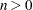. The NVAR=ALL option uses all variables in the VAR list. By default, NVAR=5.

NWITH=ALL | n

specifies the maximum number of variables in the WITH list to be displayed in the matrix plot, where. The NWITH=ALL option uses all variables in the WITH list. By default, NWITH=5.

The available scatter-options are as follows:

ALPHA=specifies thevalues for the confidence or prediction ellipses to be displayed in the scatter plots, where
. For eachvalue specified, a (
) confidence or prediction ellipse is created. By default,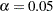.

ELLIPSE=PREDICTION | CONFIDENCE | NONE

requests prediction ellipses for new observations (ELLIPSE=PREDICTION), confidence ellipses for the mean (ELLIPSE=CONFIDENCE), or no ellipses (ELLIPSE=NONE) to be created in the scatter plots. By default, ELLIPSE=PREDICTION.

NOINSET

suppresses the default inset of summary information for the scatter plot. The inset table contains the number of observations (Observations) and correlation.

NVAR=ALL | n

specifies the maximum number of variables in the VAR list to be displayed in the plots, where. The NVAR=ALL option uses all variables in the VAR list. By default, NVAR=5.

NWITH=ALL | n

specifies the maximum number of variables in the WITH list to be displayed in the plots, where. The NWITH=ALL option uses all variables in the WITH list. By default, NWITH=5.

RANK

displays the ordered correlation coefficients for each variable. Correlations are ordered from highest to lowest in absolute value. If you specify the HOEFFDING option, thestatistics are displayed in order from highest to lowest.

SINGULAR=p

specifies the criterion for determining the singularity of a variable if you use a PARTIAL statement. A variable is considered singular if its corresponding diagonal element after Cholesky decomposition has a value less than p times the original unpartialled value of that variable. The default value is 1E8. The range of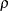is between 0 and 1.

SPEARMAN

requests a table of Spearman correlation coefficients based on the ranks of the variables. The correlations range from1 to 1. If you specify a WEIGHT statement, the SPEARMAN option is invalid.

SSCP

displays a table of the sums of squares and crossproducts. When you specify the SSCP option, the Pearson correlations will also be displayed. If you specify the OUTP= option, the output data set contains a SSCP matrix and the corresponding _TYPE_ variable value is 'SSCP.' If you use a PARTIAL statement, the unpartial SSCP matrix is displayed, and the output data set does not contain an SSCP matrix.

VARDEF=DF | N | WDF | WEIGHT | WGT

specifies the variance divisor in the calculation of variances and covariances. The default is VARDEF=DF.

Table 2.2 displays available values and associated divisors for the VARDEF= option, where n is the number of nonmissing observations, k is the number of variables specified in the PARTIAL statement, and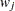is the weight associated with theth nonmissing observation.

Table 2.2 Possible Values for the VARDEF= Option

Value

Description

Divisor

DF

degrees of freedom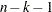N

number of observationsWDF

sum of weights minus one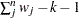WEIGHT | WGT

sum of weights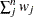Previous Page | Next Page | Top of Page